Chapter 3 Class 12 Matrices
Concept wise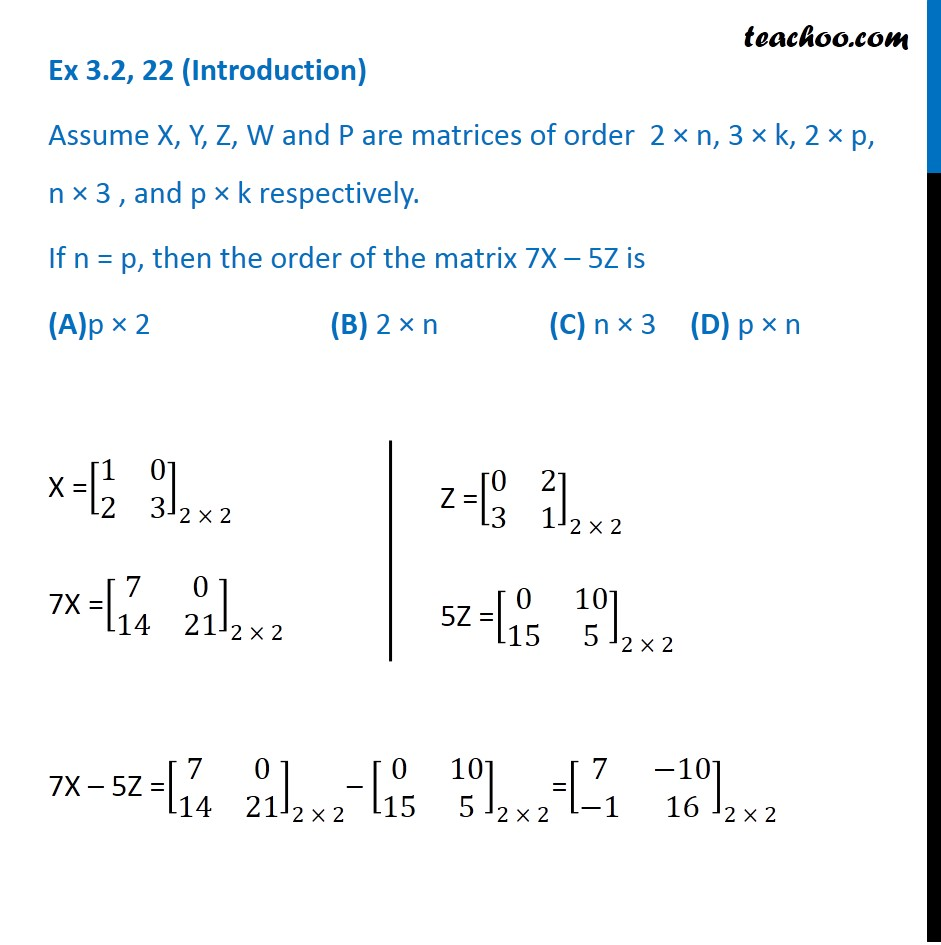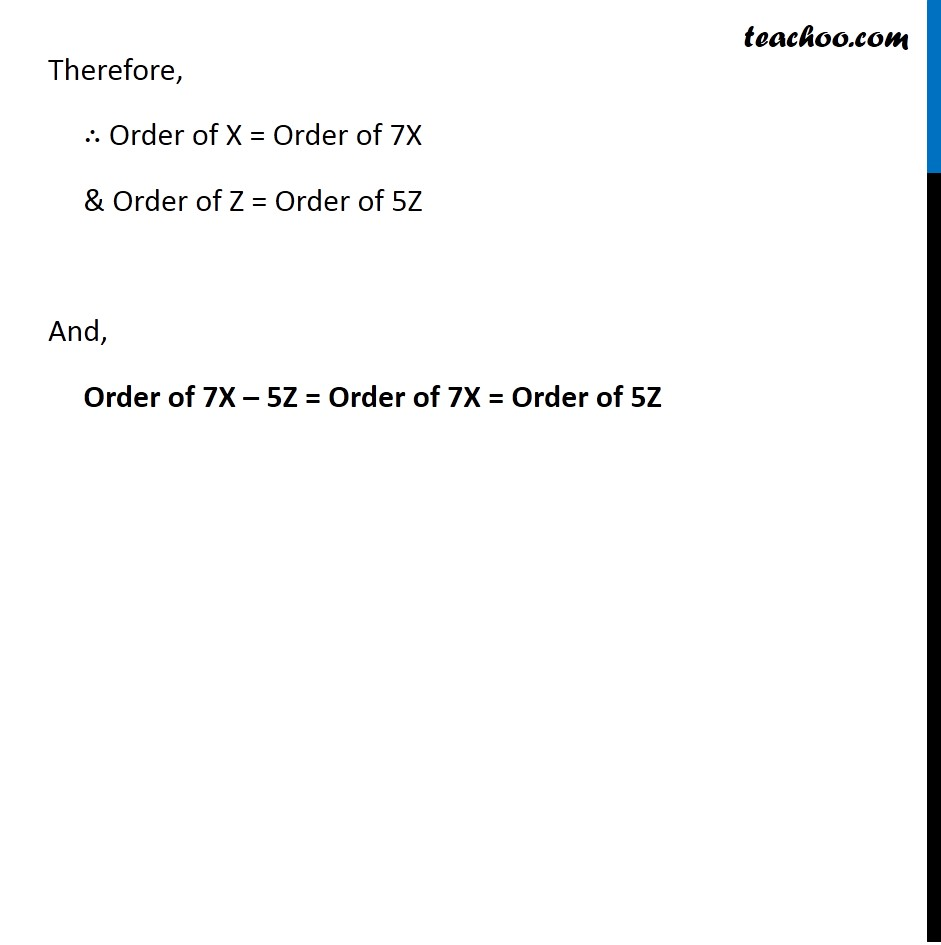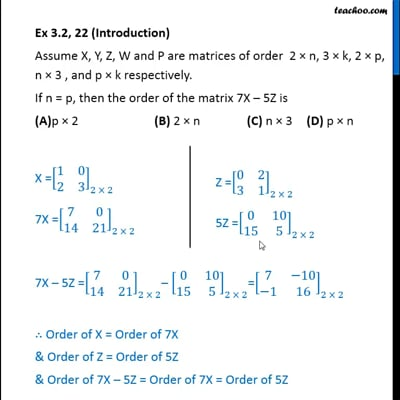This video is only available for Teachoo black users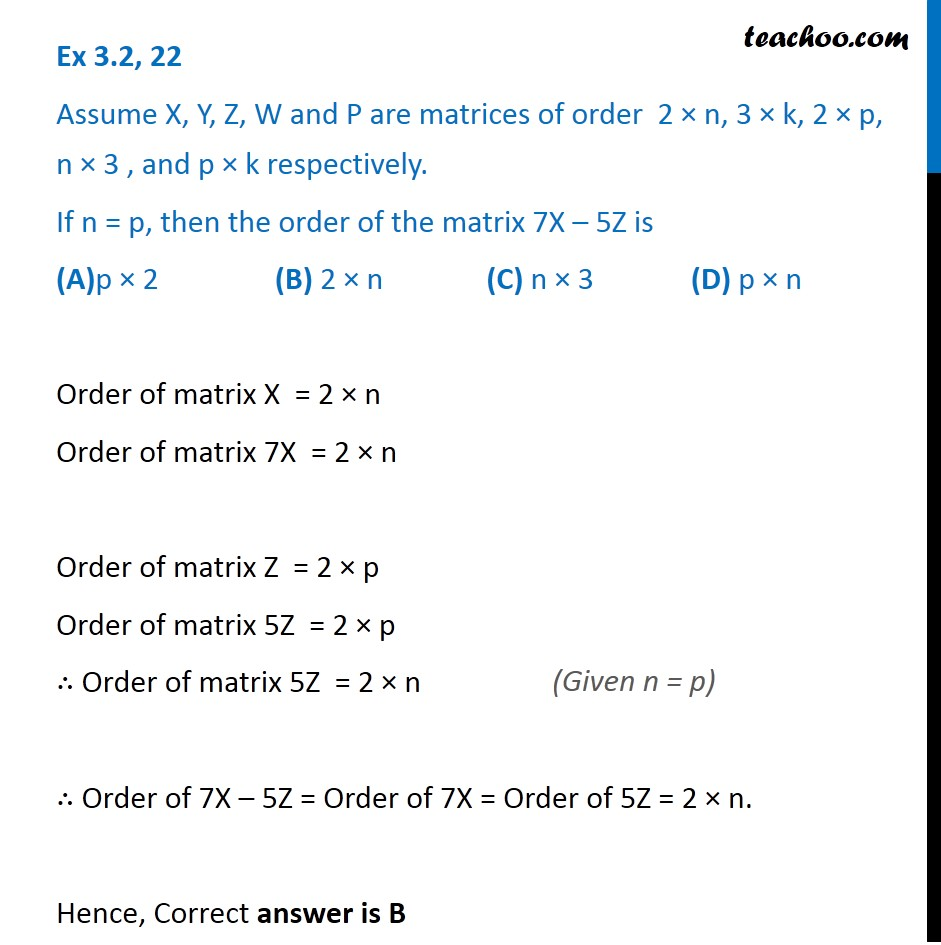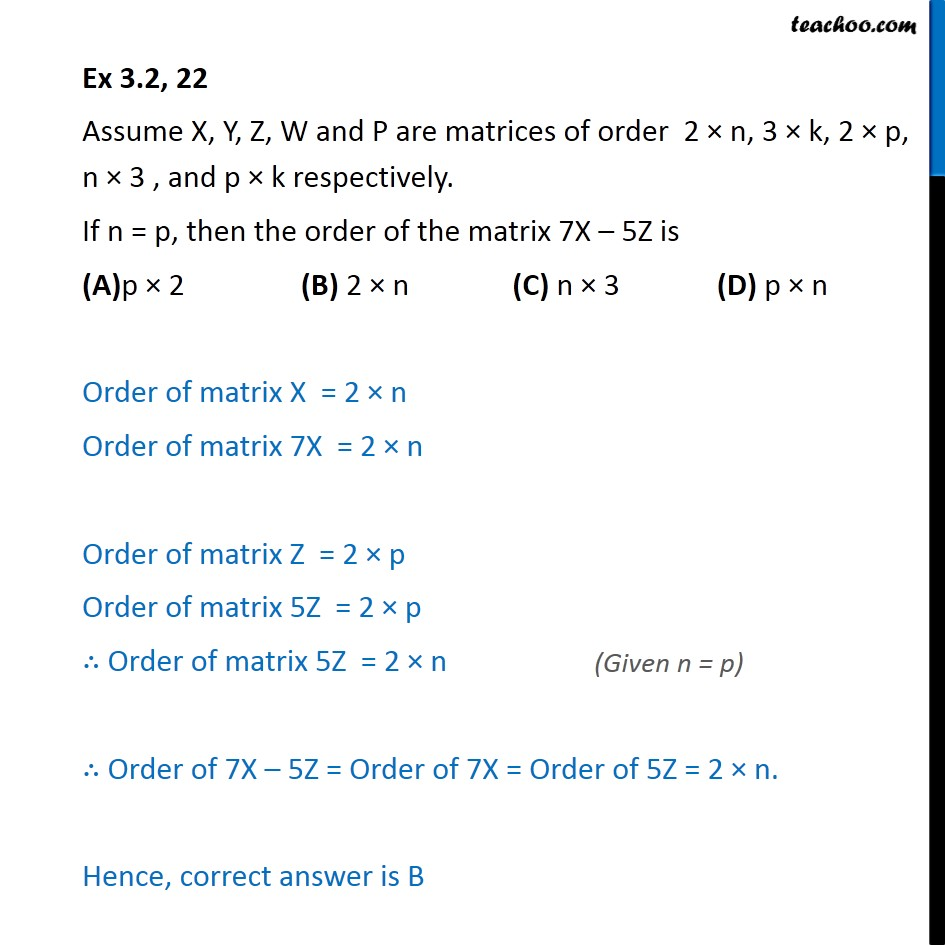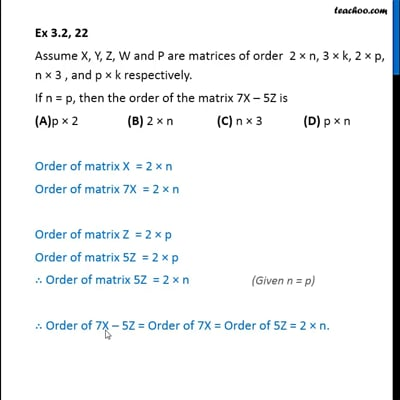This video is only available for Teachoo black users

Introducing your new favourite teacher - Teachoo Black, at only ₹83 per month

### Transcript

Ex 3.2, 22 (Introduction) Assume X, Y, Z, W and P are matrices of order 2 × n, 3 × k, 2 × p, n × 3 , and p × k respectively. If n = p, then the order of the matrix 7X – 5Z is (A)p × 2 (B) 2 × n (C) n × 3 (D) p × n X =[■8(1&0@2&3)]_(2 × 2) 7X =[■8(7&0@14&21)]_(2 × 2) 7X – 5Z =[■8(7&0@14&21)]_(2 × 2)– [■8(0&10@15&5)]_(2 × 2)=[■8(7&−10@−1&16)]_(2 × 2) ∴ Order of X = Order of 7X & Order of Z = Order of 5Z & Order of 7X – 5Z = Order of 7X = Order of 5Z Ex 3.2, 22 Assume X, Y, Z, W and P are matrices of order 2 × n, 3 × k, 2 × p, n × 3 , and p × k respectively. If n = p, then the order of the matrix 7X – 5Z is (A)p × 2 (B) 2 × n (C) n × 3 (D) p × n Order of matrix X = 2 × n Order of matrix 7X = 2 × n Order of matrix Z = 2 × p Order of matrix 5Z = 2 × p ∴ Order of matrix 5Z = 2 × n ∴ Order of 7X – 5Z = Order of 7X = Order of 5Z = 2 × n. Hence, correct answer is B